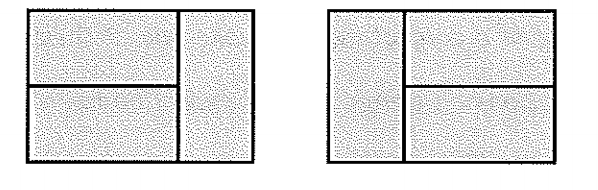$$\newcommand{\id}{\mathrm{id}}$$ $$\newcommand{\Span}{\mathrm{span}}$$ $$\newcommand{\kernel}{\mathrm{null}\,}$$ $$\newcommand{\range}{\mathrm{range}\,}$$ $$\newcommand{\RealPart}{\mathrm{Re}}$$ $$\newcommand{\ImaginaryPart}{\mathrm{Im}}$$ $$\newcommand{\Argument}{\mathrm{Arg}}$$ $$\newcommand{\norm}{\| #1 \|}$$ $$\newcommand{\inner}{\langle #1, #2 \rangle}$$ $$\newcommand{\Span}{\mathrm{span}}$$

# 1. Power of Patterns: Domino Tiling

•• Contributed by Pamini Thangarajah
• Professor (Mathematics & Computing) at Mount Royal University
$$\newcommand{\vecs}{\overset { \rightharpoonup} {\mathbf{#1}} }$$ $$\newcommand{\vecd}{\overset{-\!-\!\rightharpoonup}{\vphantom{a}\smash {#1}}}$$$$\newcommand{\id}{\mathrm{id}}$$ $$\newcommand{\Span}{\mathrm{span}}$$ $$\newcommand{\kernel}{\mathrm{null}\,}$$ $$\newcommand{\range}{\mathrm{range}\,}$$ $$\newcommand{\RealPart}{\mathrm{Re}}$$ $$\newcommand{\ImaginaryPart}{\mathrm{Im}}$$ $$\newcommand{\Argument}{\mathrm{Arg}}$$ $$\newcommand{\norm}{\| #1 \|}$$ $$\newcommand{\inner}{\langle #1, #2 \rangle}$$ $$\newcommand{\Span}{\mathrm{span}}$$ $$\newcommand{\id}{\mathrm{id}}$$ $$\newcommand{\Span}{\mathrm{span}}$$ $$\newcommand{\kernel}{\mathrm{null}\,}$$ $$\newcommand{\range}{\mathrm{range}\,}$$ $$\newcommand{\RealPart}{\mathrm{Re}}$$ $$\newcommand{\ImaginaryPart}{\mathrm{Im}}$$ $$\newcommand{\Argument}{\mathrm{Arg}}$$ $$\newcommand{\norm}{\| #1 \|}$$ $$\newcommand{\inner}{\langle #1, #2 \rangle}$$ $$\newcommand{\Span}{\mathrm{span}}$$

## Power of Patterns: Domino Tiling

### OVERVIEW & PURPOSE

Students gain an understanding of visualizing problems and explore the mathematical world of tiling.

### OBJECTIVES

1. Use a systematic approach to discover the pattern of the different tiling (on 2 x n rectangle)

2. Use knowledge of Fibonacci numbers to help create a proof

### MATERIALS NEEDED

1. Dominoes (6 dominoes per group)

2. Paper

### ACTIVITY

1. Introduce the concept of tiling to students: focusing on tiling 2 x n rectangles with dominoes. (Provide an explanation of “2 x n” and examples of how dominoes can be moved)

2. Rules:

1. There can be no gaps or overlapping on the rectangle

2. Rotating tiles to create different variations counts as another way of tiling. An example below: these are considered two different tiling1. Ask students to create a T-Table to keep track of their tiling. Labeled: ‘n’ to indicate how many dominoes they are using and ‘number of tilings’ to indicate the number of possible tiling.

2. Students will work through the table. Encourage students to come up with a formula for their answer. With prior knowledge: students will discover the sequence is the Fibonacci sequence.

### SOLVING

• Fibonacci sequence: You add the two previous numbers and continue in the same pattern. (E.g. if your first two numbers are 1 and 2, you add 1+2 = 3, which makes 3 your third number.

• Go over the various tiling patterns as a class